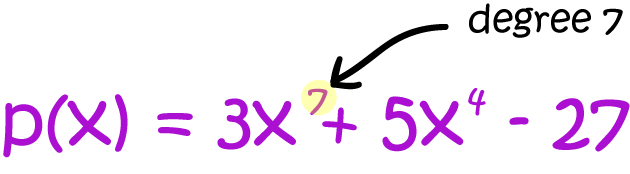# Definition of Degree (Algebra)The degree is the highest power of $x$ (or whatever the variable is) in a polynomial with one variable.

(If there is more than one variable, the degree the largest sum of all the powers involved in each term of the polynomial).

For example,

• The degree of $3x^2 + 5x + 6$ is $2$.
• The degree of $7x^{16} + 2x^2 + 23$ is $16$.
• The degree of $x^3y + 3x^2 + 2xy + 17$ is $4 = 3 + 1$.

### Description

The aim of this dictionary is to provide definitions to common mathematical terms. Students learn a new math skill every week at school, sometimes just before they start a new skill, if they want to look at what a specific term means, this is where this dictionary will become handy and a go-to guide for a studen

### Audience

Year 1 to Year 12 students

### Learning Objectives

Learn common math terms starting with letter D

Author: Subject Coach
You must be logged in as Student to ask a Question.